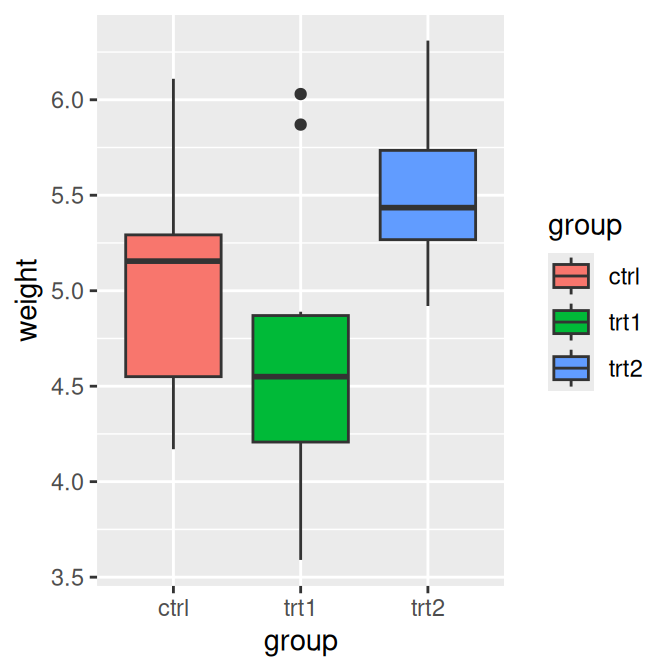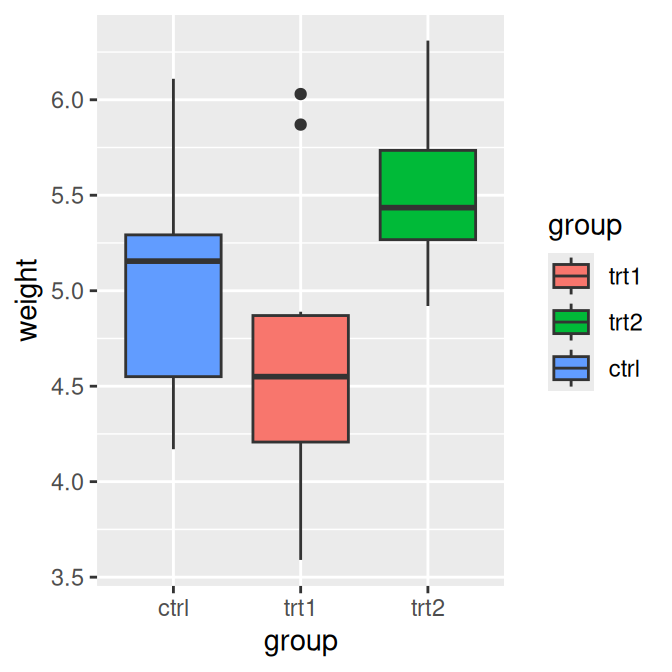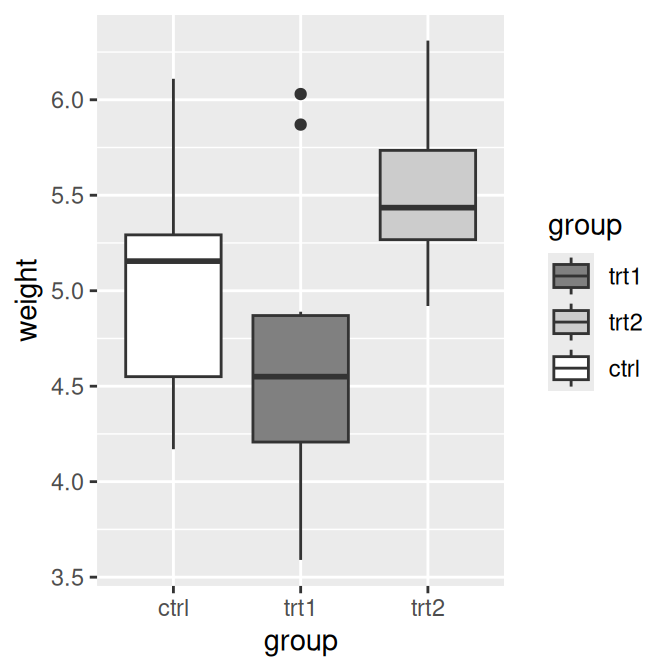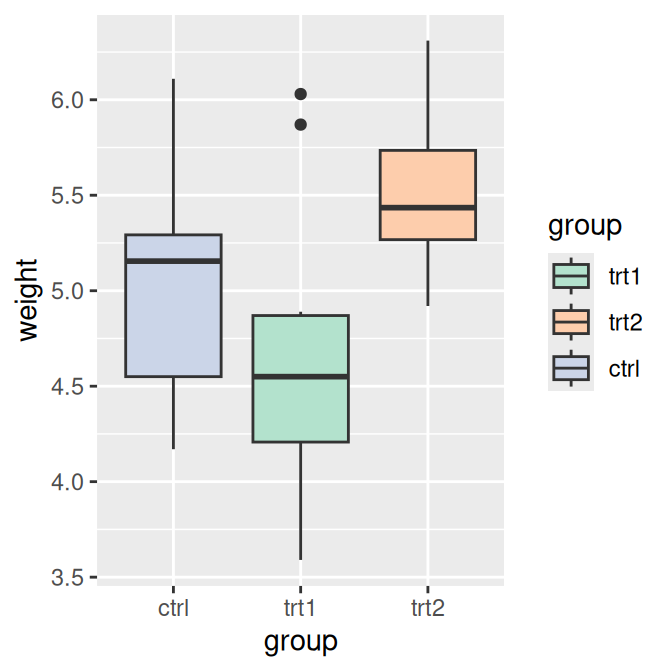## 10.3 Changing the Order of Items in a Legend

### 10.3.1 Problem

You want to change the order of the items in a legend.

### 10.3.2 Solution

Set the limits in the scale to the desired order (Figure 10.5):

``````# Create the base plot
pg_plot <- ggplot(PlantGrowth, aes(x = group, y = weight, fill = group)) +
geom_boxplot()

pg_plot

# Change the order of items
pg_plot +
scale_fill_discrete(limits = c("trt1", "trt2", "ctrl"))``````Figure 10.5: Default order for legend (left); Modified order (right)

### 10.3.3 Discussion

Note that the order of the items on the x-axis did not change. To do that, you would have to set the limits of `scale_x_discrete()` (Recipe 8.4), or change the data to have a different factor level order (Recipe 15.8).

In the preceding example, group was mapped to the fill aesthetic. By default this uses `scale_fill_discrete()` (which is the sameas `scale_fill_hue()`), which maps the factor levels to colors that are equally spaced around the color wheel. We could have used a different `scale_fill_``xxx``()`, though. For example, we could use a grey palette (Figure 10.6, left):

``````pg_plot +
scale_fill_grey(start = .5, end = 1, limits = c("trt1", "trt2", "ctrl"))``````

Or we could use a palette from RColorBrewer (Figure 10.6, right):

``````pg_plot +
scale_fill_brewer(palette = "Pastel2", limits = c("trt1", "trt2", "ctrl"))``````Figure 10.6: Modified order with a grey palette (left); With a palette from RColorBrewer (right)

All the previous examples were for fill. If you use scales for other aesthetics, such as colour (for lines and points) or shape (for points), you must use the appropriate scale. Commonly used scales include:

• `scale_fill_discrete()`
• `scale_fill_hue()`
• `scale_fill_manual()`
• `scale_fill_grey()`
• `scale_fill_brewer()`
• `scale_colour_discrete()`
• `scale_colour_hue()`
• `scale_colour_manual()`
• `scale_colour_grey()`
• `scale_colour_brewer()`
• `scale_shape_manual()`
• `scale_linetype()`

By default, using `scale_fill_discrete()` is equivalent to using `scale_fill_hue()`; the same is true for color scales.